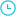# Totally Cool Math: Multiplication

SKU:

\$19.9520 Minutes - Color

- +

Categories: Elementary Films,

• Description

With this video you will learn:
- using end zeros
- double & doubling doubles
- multiplying by 11
- halving & doubling
- subtraction method
- criss-cross multiplication
- compatible factors

Part of the "Totally Cool Math" series, an innovative program designed by teachers that teaches mental math and makes learning easier, faster, and fun!

96 minutes • Color

--

With this video you will learn:
- using end zeros
- double & doubling doubles
- multiplying by 11
- halving & doubling
- subtraction method
- criss-cross multiplication
- compatible factors

Part of the "Totally Cool Math" series, an innovative program designed by teachers that teaches mental math and makes learning easier, faster, and fun!

96 minutes • Color

With this video you will learn:
- using end zeros
- double & doubling doubles
- multiplying by 11
- halving & doubling
- subtraction method
- criss-cross multiplication
- compatible factors

Part of the "Totally Cool Math" series, an innovative program designed by teachers that teaches mental math and makes learning easier, faster, and fun!

96 minutes • Color

--

With this video you will learn:
- using end zeros
- double & doubling doubles
- multiplying by 11